Next: Example 12,2: Refraction Up: Geometric Optics Previous: Worked Examples

## Example 12.1: The corner-cube reflector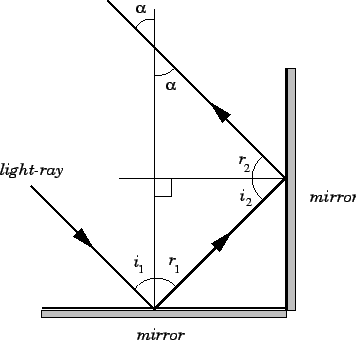Question: Two mirrors are placed at right-angles to one another. Show that a light-ray incident from any direction in the plane perpendicular to both mirrors is reflected through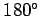.

Answer: Consider the diagram. We are effectively being asked to prove that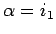, for any value of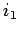. Now, from trigonometry,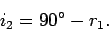But, from the law of reflection,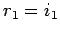and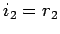, so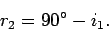Trigonometry also yields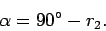It follows from the previous two equations that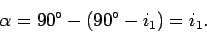Hence,, for all values of.

It can easily be appreciated that a combination of three mutually perpendicular mirrors would reflect a light-ray incident from any direction through. Such a combination of mirrors is called a corner-cube reflector. Astronauts on the Apollo 11 mission (1969) left a panel of corner-cube reflectors on the surface of the Moon. These reflectors have been used ever since to measure the Earth-Moon distance via laser range finding (basically, a laser beam is fired from the Earth, reflects off the corner-cube reflectors on the Moon, and then returns to the Earth. The time of travel of the beam can easily be converted into the Earth-Moon distance). The Earth-Moon distance can be measured to within an accuracy of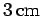using this method.Next: Example 12,2: Refraction Up: Geometric Optics Previous: Worked Examples
Richard Fitzpatrick 2007-07-14